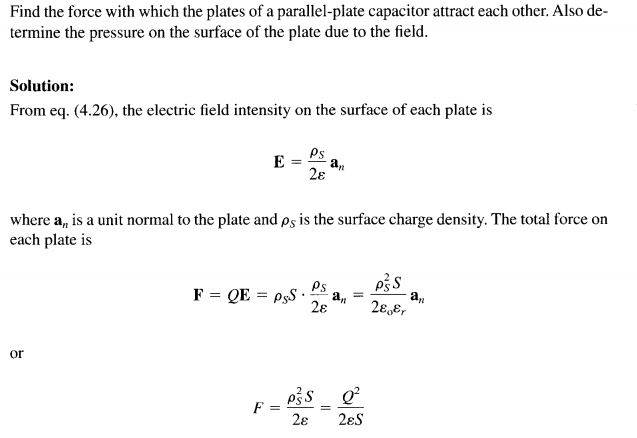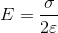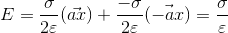# Electric filed of each plate of capacitor

hello
here in this problem as you see we want to obtain the pressure between thew plate of capacitormy question is about the capacitor each plate electric filed equation.
as we know the electric filed of an infinite plate isbut the equation of an conductor plate is

as we know the each plate of capacitor is conductor.why in above problem the write use the infinite plate equation instead of conductor plate equation?

Thanks

ehild
Homework Helper
The magnitude of the electric field between the capacitor plates is E=ρs/ε. You can follow an other approach, based on potential energy.

Assume that the capacitor plates are at distance x from each other and the area of the plates is S. One plate is earthed, and you move charges from the grounded plate to the other one. Initially no charge is on the other plate, you do not need any work. I there is q charge on the plate, the electric field is q/(Sε) and you do dW=Exdq work to transfer dq charge. So the net work when the capacitor is charged to Q is
$$W=\int_0^Q{\frac{x}{Sε}qdq}=\frac{x}{2Sε}Q^2$$ That work is the potential energy of the capacitor. Its negative gradient is the force between the capacitor plates. $$F=-\frac{Q^2}{2Sε}$$. The negative sign means that the force opposes the motion of the capacitor plate.

ehild

•1 person
Thanks ehild
but my question is something else.
yes, if we take the sum of two electric filed with surface electric filed equationbut my question is why the writer use surface electric filed equation instead of conductor electric filed for each plate?because as we know and prove the electric filed of conductor is
http://hyperphysics.phy-astr.gsu.edu/hbase/electric/gausur.html

ehild
Homework Helper
The electric field inside the capacitor is Q/(εS). The surface charge density is Q/S on one plate and -Q/S on the other plate. But that electric field has been created by the charges on both plates, and the charges do not act on themselves. So you need to take the field of one plate and the force of that field on the charge of the other plate. I prefer the derivation with the energy.

ehild

Thanks ehlid
now i change and say better my new question: the electric filed of capacitor and not force between two plate.
as i know we can use superposition to maintain the electric filed of between two conductor of capacitor.
so the electric filed of each conductor surface is
that an is perpendicular unit vector .so we assume that positive charge on the top surface and negative charge on the bottom surface.
so we want to obtain the electric filed between two surface.
top surface:
bottom surface:

and superposition:
Et=E1+E2

lightgrav
Homework Helper
You saw the downward E , below the (+) top plate,
add to the downward E , above the (-) top plate.
They only add to each other in between the plates
... they cancel above the top plate and below the bottom plate.
That is one way to obtain this "between-the-plates" formula;
use Gauss' Law with clever geometric symmetry, not nasty integrations.

•1 person